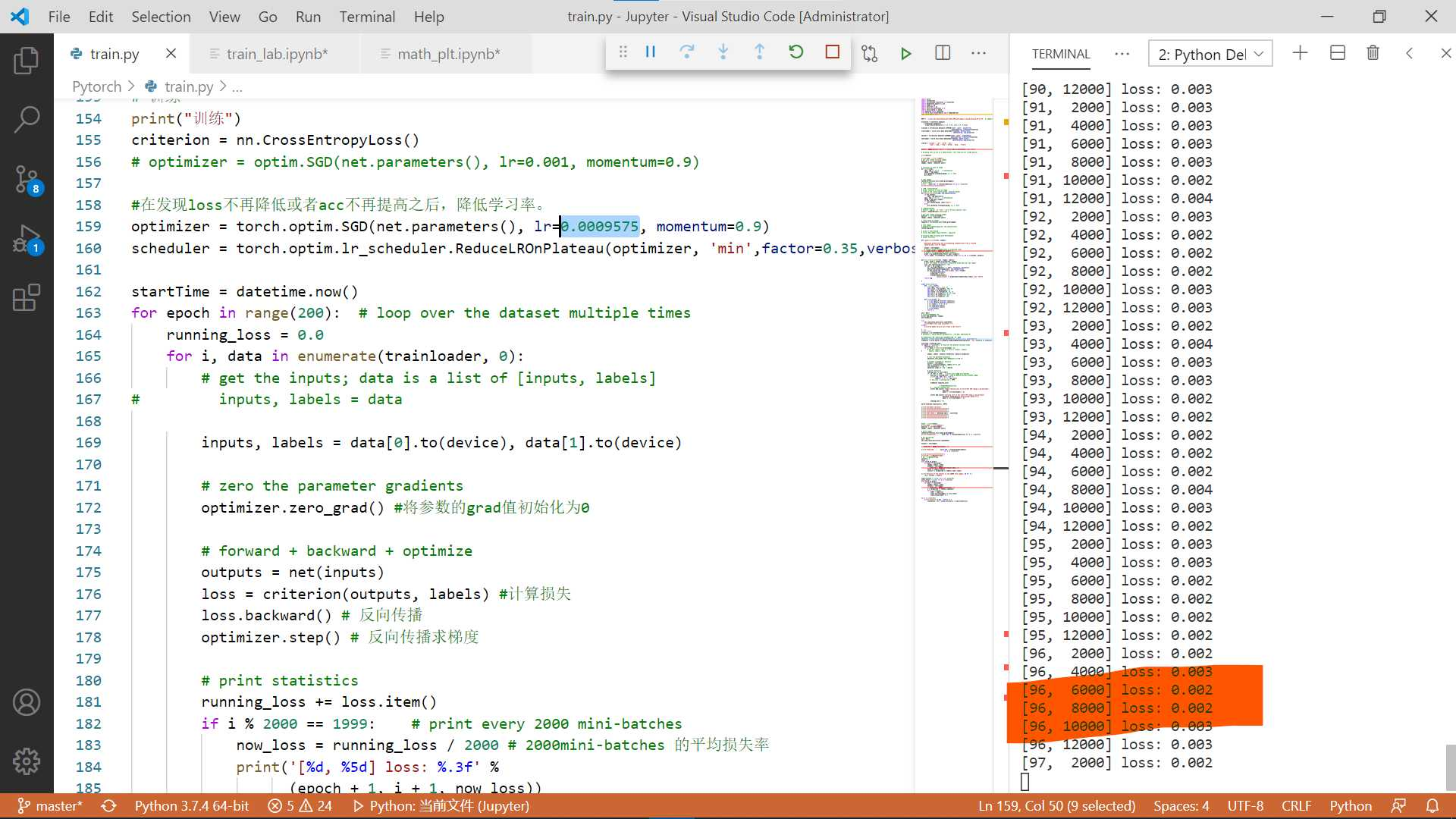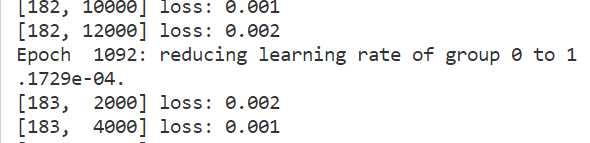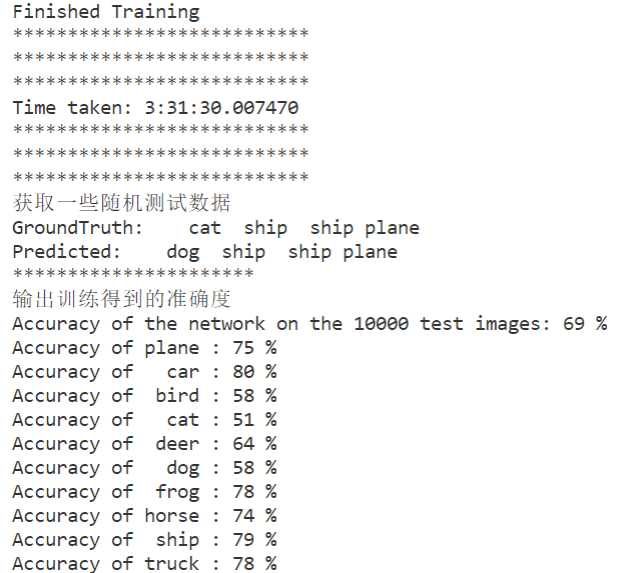# Pytorch using ReduceLROnPlateau to update learning rates

The Post Created(Updated) On 04/22/2022，Please note the timeliness of the article!# Reason

I wrote a Pytorch learning rate update, which feels based on whether the number of times the loss rises or falls to dynamically update the learning rate, I think it’s a fun thing. I got it wrong for a long time, but I got it today!

# Analysis

## Explanation

• ReduceLROnPlateau(optimizer, mode=’min’, factor=0.1, patience=10, verbose=False, threshold=0.0001, threshold_ mode=’rel’, cooldown=0, min_lr=0, eps=1e-08)

Decrease the learning rate after finding that loss is no longer decreasing or acc is no longer increasing. The meaning of each parameter is as follows.

Parameters Meaning
mode ‘min’ mode detects if the metric is no longer decreasing, and ‘max’ mode detects if the metric is no longer increasing;
factor the trigger condition after lr*=factor;
patience the cumulative number of times the metric no longer decreases (or increases);
verbose print after the trigger condition;
threshold focus only on significant changes above the threshold;
threshold_mode There are two threshold calculation modes, rel and abs. rel rule: max mode is significant if it exceeds best(1+threshold), min mode is significant if it is below best(1-threshold); abs rule: max mode is significant if it exceeds best+ threshold for significant, min mode if lower than best-threshold for significant;
cooldown after triggering the condition once, wait for a certain epoch before testing to avoid lr falling too fast;
min_lr the minimum allowed lr;
eps If the difference between the old and new lr is smaller than 1e-8, then this update is ignored. |
• Example, as shown in the figure the y-axis is lr,x is the order of adjustment, the initial learning rate is 0.0009575
Then the equation of learning rate is: lr = 0.0009575 * (0.35)^x
import math
import matplotlib.pyplot as plt
#%matplotlib inline

x = 0
o = []
p = []
o.append(0)
p.append(0.0009575)
while(x < 8):
x += 1
y = 0.0009575 * math.pow(0.35,x)
o.append(x)
p.append(y)
print('%d: %.50f' %(x,y))

plt.plot(o,p,c='red',label='test') # corresponding data for x,y axis,c:color,label respectively
plt.legend(loc='best') # show the label, loc is the display position (best is the position the system thinks is best)
plt.show()


## Difficult point

The first is the initial learning rate (I am currently in contact with the miniest and the following image classification seems to be 0.001, I realized that I set the training adjustment for 0.0009575, this value is the last experiment forgot to change, but found good results, the first run the code close to 0.001 so small (the loss value), which is difficult to estimate the product factor as well as the judgment that says how many times there is no decrease (increase) after the decision to transform the learning rate. My own best approach is to first train at the default constant 0.001 (combined with tensoarboard ) to observe where the problem starts to occur from here Determine the number of times, and the product coefficient, I personally feel that it is better to use the above code to get a smoother and very small change in the number to use as a choice. It is recommended that when doing such tests you can back up the model first to avoid wasting too much time!

# Example

• The initial learning rate of the example is 0.0009575, the coefficient of the product term is: 0.35, in my example x change condition is: cumulative 125 times without reduction then x plus 1; own training after the first lr change (from 0.0009575 change to 0.00011729) loss value slowly oriented to 0.001 (as shown in the first graph), the accuracy rate reached 69% ;.import torch
import torchvision
import torchvision.transforms as transforms
import matplotlib.pyplot as plt
import numpy as np
import torch.nn as nn
import torch.nn.functional as F
import torch.optim as optim
from datetime import datetime
from torch.utils.tensorboard import SummaryWriter
from torch.optim import *

PATH = './cifar_net_tensorboard_net_width_200_and_chang_lr_by_decrease_0_35^x.pth'  # 保存模型地址

transform = transforms.Compose(
[transforms.ToTensor(),
transforms.Normalize((0.5, 0.5, 0.5), (0.5, 0.5, 0.5))])

trainset = torchvision.datasets.CIFAR10(root='./data', train=True,
shuffle=True, num_workers=0)

testset = torchvision.datasets.CIFAR10(root='./data', train=False,
shuffle=False, num_workers=0)

classes = ('plane', 'car', 'bird', 'cat',
'deer', 'dog', 'frog', 'horse', 'ship', 'truck')

device = torch.device("cuda:0" if torch.cuda.is_available() else "cpu")

# Assuming that we are on a CUDA machine, this should print a CUDA device:

print(device)

print("获取一些随机训练数据")
# get some random training images
images, labels = dataiter.next()

# functions to show an image
def imshow(img):
img = img / 2 + 0.5     # unnormalize
npimg = img.numpy()
plt.imshow(np.transpose(npimg, (1, 2, 0)))
plt.show()

# show images
imshow(torchvision.utils.make_grid(images))
# print labels
print(' '.join('%5s' % classes[labels[j]] for j in range(4)))
print("**********************")

# helper function to show an image
# (used in the plot_classes_preds function below)
def matplotlib_imshow(img, one_channel=False):
if one_channel:
img = img.mean(dim=0)
img = img / 2 + 0.5     # unnormalize
npimg = img.cpu().numpy()
if one_channel:
plt.imshow(npimg, cmap="Greys")
else:
plt.imshow(np.transpose(npimg, (1, 2, 0)))

# 设置tensorBoard
# default log_dir is "runs" - we'll be more specific here
writer = SummaryWriter('runs/train')

# get some random training images
images, labels = dataiter.next()

# create grid of images
img_grid = torchvision.utils.make_grid(images)

# show images
# matplotlib_imshow(img_grid, one_channel=True)
imshow(img_grid)

# write to tensorboard

# Tracking model training with TensorBoard
# helper functions

def images_to_probs(net, images):
'''
Generates predictions and corresponding probabilities from a trained
network and a list of images
'''
output = net(images)
# convert output probabilities to predicted class
_, preds_tensor = torch.max(output, 1)
# preds = np.squeeze(preds_tensor.numpy())
preds = np.squeeze(preds_tensor.cpu().numpy())
return preds, [F.softmax(el, dim=0)[i].item() for i, el in zip(preds, output)]

def plot_classes_preds(net, images, labels):
preds, probs = images_to_probs(net, images)
# plot the images in the batch, along with predicted and true labels
fig = plt.figure(figsize=(12, 48))
for idx in np.arange(4):
ax = fig.add_subplot(1, 4, idx+1, xticks=[], yticks=[])
matplotlib_imshow(images[idx], one_channel=True)
ax.set_title("{0}, {1:.1f}%\n(label: {2})".format(
classes[preds[idx]],
probs[idx] * 100.0,
classes[labels[idx]]),
color=("green" if preds[idx]labels[idx].item() else "red"))
return fig

#

class Net(nn.Module):
def __init__(self):
super(Net, self).__init__()
self.conv1 = nn.Conv2d(3, 200, 5)
self.pool = nn.MaxPool2d(2, 2)
self.conv2 = nn.Conv2d(200, 16, 5)
self.fc1 = nn.Linear(16 * 5 * 5, 120)
self.fc2 = nn.Linear(120, 84)
self.fc3 = nn.Linear(84, 10)

def forward(self, x):
x = self.pool(F.relu(self.conv1(x)))
x = self.pool(F.relu(self.conv2(x)))
x = x.view(-1, 16 * 5 * 5)
x = F.relu(self.fc1(x))
x = F.relu(self.fc2(x))
x = self.fc3(x)
return x

net = Net()
# # 把net结构可视化出来
net.to(device)

try:
except:
print("no model file,it will creat a new file!")

# 训练
print("训练")
criterion = nn.CrossEntropyLoss()
# optimizer = optim.SGD(net.parameters(), lr=0.001, momentum=0.9)

#在发现loss不再降低或者acc不再提高之后，降低学习率。
optimizer = torch.optim.SGD(net.parameters(), lr=0.0009575, momentum=0.9)
scheduler = torch.optim.lr_scheduler.ReduceLROnPlateau(optimizer, 'min',factor=0.35,verbose=1,min_lr=0.0001,patience=125)

startTime = datetime.now()
for epoch in range(200):  # loop over the dataset multiple times
running_loss = 0.0
for i, data in enumerate(trainloader, 0):
# get the inputs; data is a list of [inputs, labels]
#         inputs, labels = data

inputs, labels = data.to(device), data.to(device)

# forward + backward + optimize
outputs = net(inputs)
loss = criterion(outputs, labels) #计算损失
loss.backward() # 反向传播
optimizer.step() # 反向传播求梯度

# print statistics
running_loss += loss.item()
if i % 2000  1999:    # print every 2000 mini-batches
now_loss = running_loss / 2000 # 2000mini-batches 的平均损失率
print('[%d, %5d] loss: %.3f' %
(epoch + 1, i + 1, now_loss))
# now_loss = running_loss / 2000

scheduler.step(now_loss)

# 把数据写入tensorflow
# ...log the running loss
writer.add_scalar('image training loss on net width 200 chang_lr_by_decrease',
now_loss,

writer.add_scalar('learning rate on net width 200 chang_lr_by_decrease',
optimizer.state_dict()['param_groups']['lr'],

running_loss = 0.0

torch.save(net.state_dict(), PATH)

print('Finished Training')
print("***************************")
print("***************************")
print("***************************")
print("Time taken:", datetime.now() - startTime)
print("***************************")
print("***************************")
print("***************************")

#获取一些随机测试数据
print("获取一些随机测试数据")
images, labels = dataiter.next()

# print images
imshow(torchvision.utils.make_grid(images))
print('GroundTruth: ', ' '.join('%5s' % classes[labels[j]] for j in range(4)))

# 恢复模型并测试
net = Net()

outputs = net(images)

_, predicted = torch.max(outputs, 1)

print('Predicted: ', ' '.join('%5s' % classes[predicted[j]]
for j in range(4)))

print("**********************")
print("输出训练得到的准确度")
# 输出训练得到的准确度
correct = 0
total = 0
images, labels = data
outputs = net(images)
_, predicted = torch.max(outputs.data, 1)
total += labels.size(0)
correct += (predicted  labels).sum().item()

print('Accuracy of the network on the 10000 test images: %d %%' % (
100 * correct / total))

class_correct = list(0. for i in range(10))
class_total = list(0. for i in range(10))
images, labels = data
outputs = net(images)
_, predicted = torch.max(outputs, 1)
c = (predicted  labels).squeeze()
for i in range(4):
label = labels[i]
class_correct[label] += c[i].item()
class_total[label] += 1

for i in range(10):
print('Accuracy of %5s : %2d %%' % (
classes[i], 100 * class_correct[i] / class_total[i]))


https://blog.emperinter.info/2022/04/22/pytorch-using-reducelronplateau-to-update-learning-rates